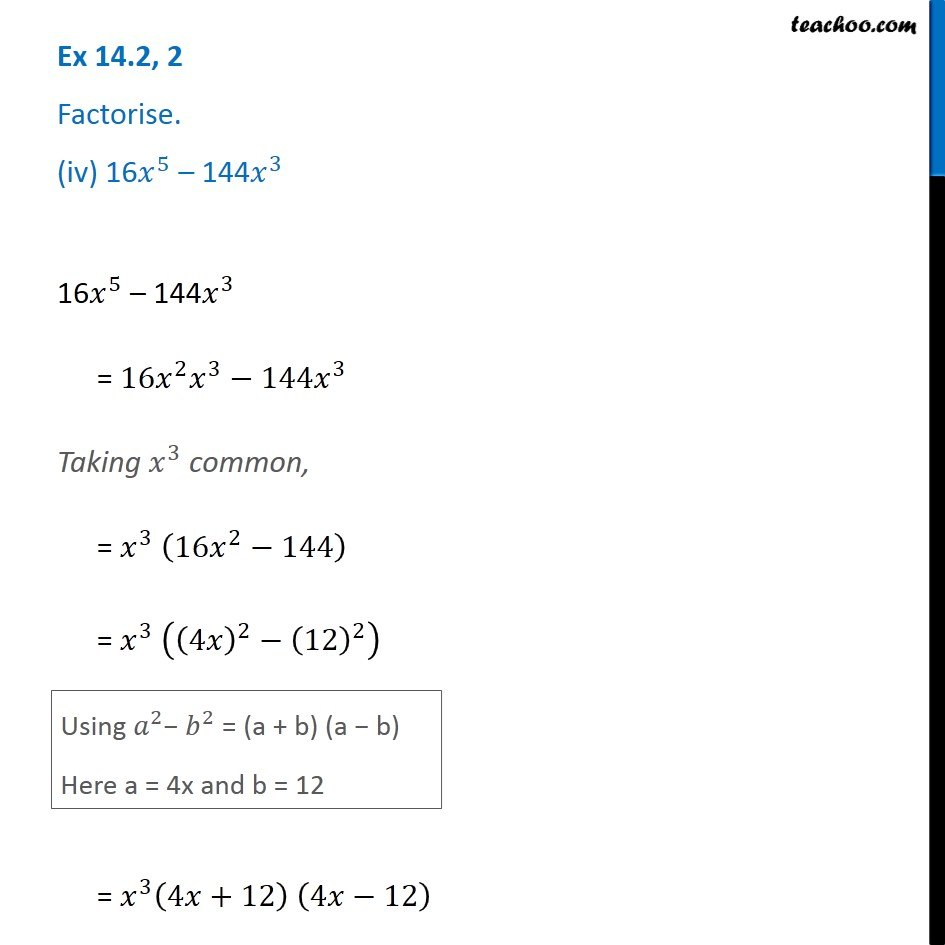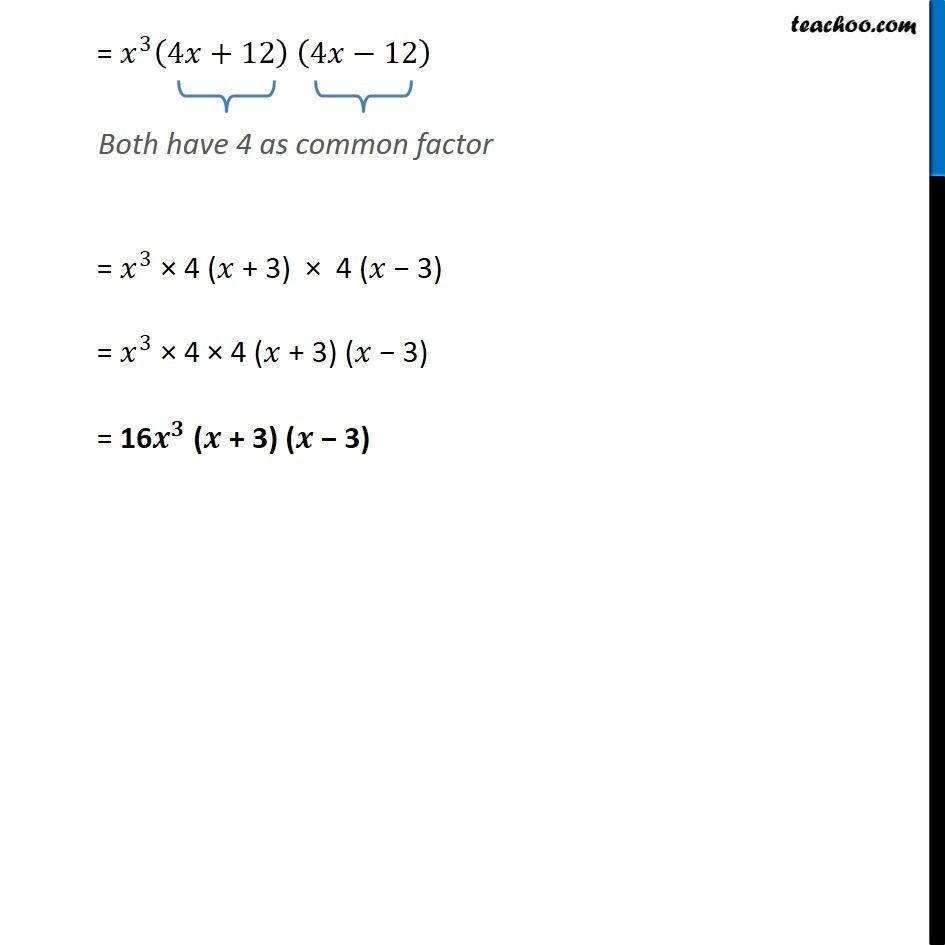Subscribe to our Youtube Channel - https://you.tube/teachoo

1. Chapter 14 Class 8 Factorisation
2. Concept wise
3. Factorisation using identities

Transcript

Ex 14.2, 2 Factorise. (iv) 16𝑥^5 – 144𝑥^3 16𝑥^5 – 144𝑥^3 = 〖16𝑥〗^2 𝑥^3−144𝑥^3 Taking 𝑥^3 common, = 𝑥^3 (16𝑥^2−144) = 𝑥^3 ((4𝑥)^2−(12)^2 ) Using 𝑎^2− 𝑏^2 = (a + b) (a − b) Here a = 4x and b = 12 = 𝑥^3 (4𝑥+12) (4𝑥−12) Both have 4 as common factor = 𝑥^3 × 4 (𝑥 + 3) × 4 (𝑥 − 3) = 𝑥^3 × 4 × 4 (𝑥 + 3) (𝑥 − 3) = 16𝒙^𝟑 (𝒙 + 3) (𝒙 − 3)

Factorisation using identities